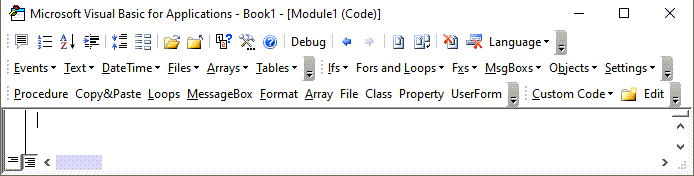# VBA InStrRev Function – Find Text From Right

This tutorial will demonstrate how to use the InStrRev VBA function to find text from the right..

## InStrRev Function

The VBA InStrRev function searches for the position of a substring inside a string. It returns the position counting from left to right but it performs the search from the end of the string to the beginning.

### VBA InStrRev Find Substring

The VBA InStrRev function can be used the find the position of a substring inside a string.

``````Sub InStrRevExample_1()
MsgBox InStrRev("ABCABC", "C")           'Result is: 6
MsgBox InStrRev("ABCABC", "BC")          'Result is: 5
MsgBox InStrRev("La La Land", "L")       'Result is: 7
MsgBox InStrRev("La La Land", "La")      'Result is: 7
MsgBox InStrRev("La La Land", "La ")     'Result is: 4
MsgBox InStrRev("La La Land", "M")       'Result is: 0
End Sub
``````

### VBA InStrRev Starting Position

VBA InStrRev Function can start the search for a substring from an assigned position counted from left to right.

``````Sub InStrRevExample_2()
MsgBox InStrRev("La La Land", "L")         'Result is: 7
MsgBox InStrRev("La La Land", "L", 8)      'Result is: 7
MsgBox InStrRev("La La Land", "L", 7)      'Result is: 7
MsgBox InStrRev("La La Land", "L", 6)      'Result is: 4
MsgBox InStrRev("La La Land", "L", 4)      'Result is: 4
MsgBox InStrRev("La La Land", "L", 3)      'Result is: 1
End Sub
``````

### VBA InStrRev Case Sensitivity

VBA InStrRev Function by default is case sensitive. You can change that behavior to case insensitive using an optional parameter. In that case, you must also define the starting position of the search.

``````Sub InStrRevExample_3()
'InstrRev by default is case sensitive
MsgBox InStrRev("La La Land", "L")     'Result is: 7
MsgBox InStrRev("La La Land", "l")     'Result is: 0

'InstrRev can perform case insensitive searches
MsgBox InStrRev("La La Land", "L", -1, vbTextCompare)     'Result is: 7
MsgBox InStrRev("La La Land", "l", -1, vbTextCompare)     'Result is: 7
End Sub
``````

### VBA InStrRev Last Space

VBA InStrRev Function can find the position of the last space in a string.

``````Sub InStrRevExample_4()
MsgBox InStrRev("La La Land", " ")
'Result is: 6
MsgBox InStrRev("Leonardo da Vinci", " ")
'Result is: 12
MsgBox InStrRev("May the Force be with you", " ")
'Result is: 22

End Sub
``````

### VBA InStrRev Second to Last Space

VBA InStrRev function can find the position of the second to last space in a string. We can use VBA InStrRev function to find the last space in a string and then we can use again InStrRev with a new starting point to find the position of the second to last space

``````Sub InStrRevExample_5()
Dim LastPos As Integer

LastPos = InStrRev("May the Force be with you", " ")
MsgBox LastPos 'Result is: 22

Dim SecondLastPos As Integer

SecondLastPos = InStrRev("May the Force be with you", " ", LastPos - 1)
MsgBox SecondLastPos 'Result is: 17
'InStrRev started the search for space just before the last space
'It will find the second to last space because it searches from right to left
End Sub
``````

Stop searching for VBA code online. Learn more about AutoMacro - A VBA Code Builder that allows beginners to code procedures from scratch with minimal coding knowledge and with many time-saving features for all users!### VBA InStrRev Return Filename

VBA InStrRev function can be used to easily return the filename from a string that contains the path and the filename. We will use InStrRev function with functions VBA Len and VBA Right.

VBA Len function returns the length of a string:

``MsgBox Len("XBCX")      'Result is: 4``

VBA Right function returns n characters from the end of a string:

``MsgBox Right("ABCDE", 2)      'Result is: DE``

We can use InStrRev function to find the last occurrence of “\” in the pathname and use Len function to calculate the length of the filename. Right can then extract the filename.

``````Sub InStrRevExample_4()
Dim PathEx As String
PathEx = "C:\MyFiles\Other\UsefulFile.pdf"

Dim FilenameEx As String

FilenameEx = Right(PathEx, Len(PathEx) - InStrRev(PathEx, "\"))
MsgBox FilenameEx 'Result is: UsefulFile.pdf

'Lets break down this code
MsgBox Len(PathEx)
'This is the length of the path name (31)

MsgBox InStrRev(PathEx, "\")
'This is the position of the last \ in string

MsgBox Len(PathEx) - InStrRev(PathEx, "\")
'This is the length of the filename (14)
'The difference between the full length and the position of the last \ in string
'(31-27=14)

'Now we know the length of the filename and of course it is at the end
'Right function is ideal for this situation
FilenameEx = Right(PathEx, Len(PathEx) - InStrRev(PathEx, "\"))

End Sub``````